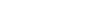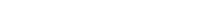having four straight equal sides and four angles of 90° - square, a flat shape with four straight sides, two of which are longer than the other two, and four angles of 90° - rectangle, a flat shape with eight straight sides and eight angles - octagon, a flat shape with three straight sides and three angles - triangle, a flat shape with six straight sides and six angles - hexagon, a flat shape with five straight sides and five angles - pentagon, a flat shape with seven straight sides and seven angles - heptagon, a flat shape with four straight sides, one pair of opposite sides being parallel and the other pair not parallel - trapezium, a flat shape with four equal sides and four angles which are not 90° - rhombus, a flat shape with six straight sides and six angles - hexagon, ​like an egg in shape - oval, a flat shape with four straight sides, the opposite sides being the same distance apart at every point and equal to each other - parallelogram, one half of a circle - semicircle, a flat shape with ten straight sides and ten angles - decagon, a flat shape with nine straight sides and nine angles - nonagon, a completely round flat shape - circle,

# English via Engineering U2

Flash cards is an open-ended template. It does not generate scores for a leaderboard.

### Options### Switch template

#### Interactives